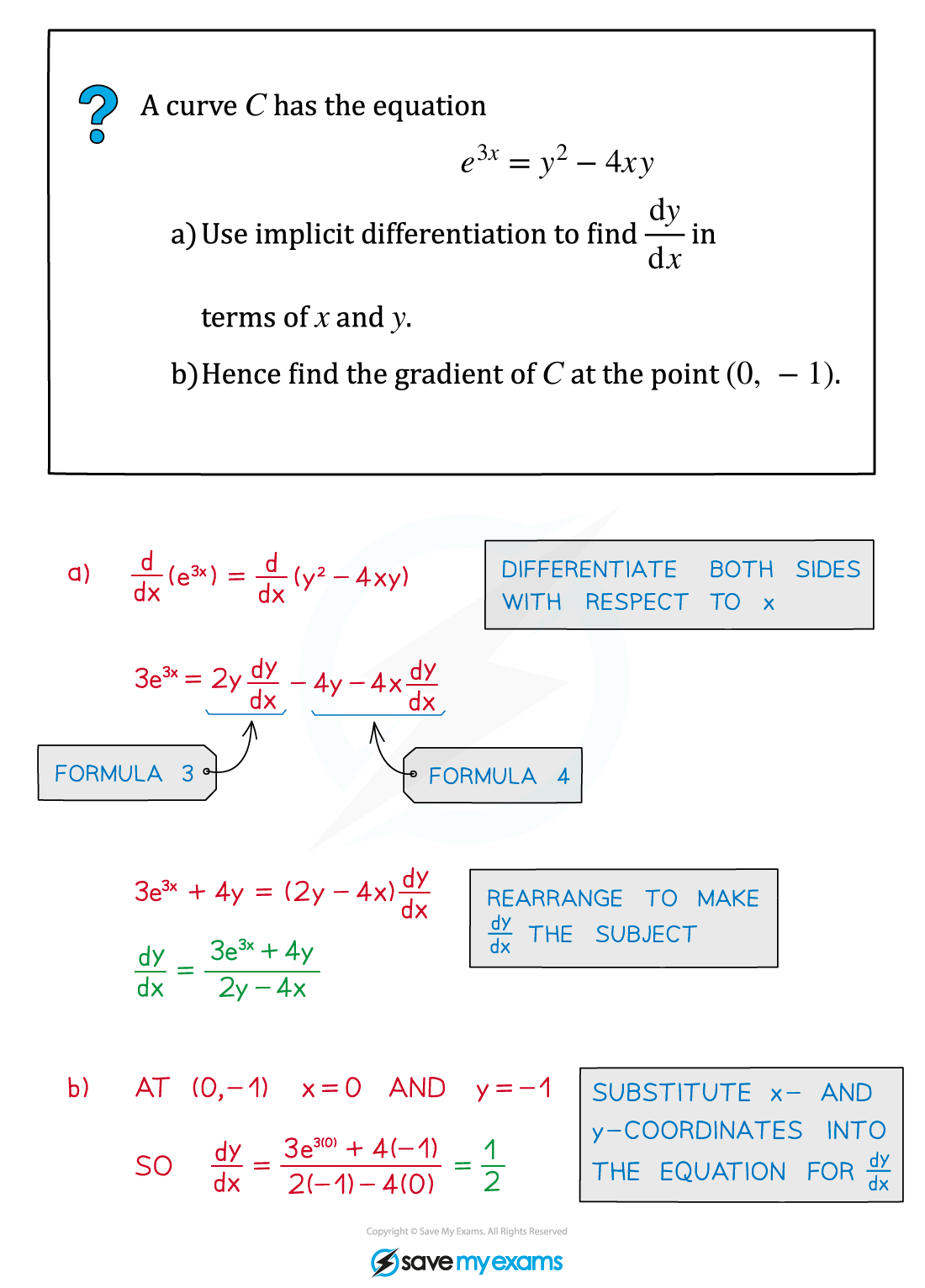# AQA A Level Maths: Pure复习笔记7.5.1 Implicit Differentiation

### Implicit Differentiation

#### What is implicit differentiation?

• An equation connecting x and y is not always easy to write explicitly in the form y= f(x) or x = f(y)
• However you can still differentiate such an equation implicitly using the chain rule: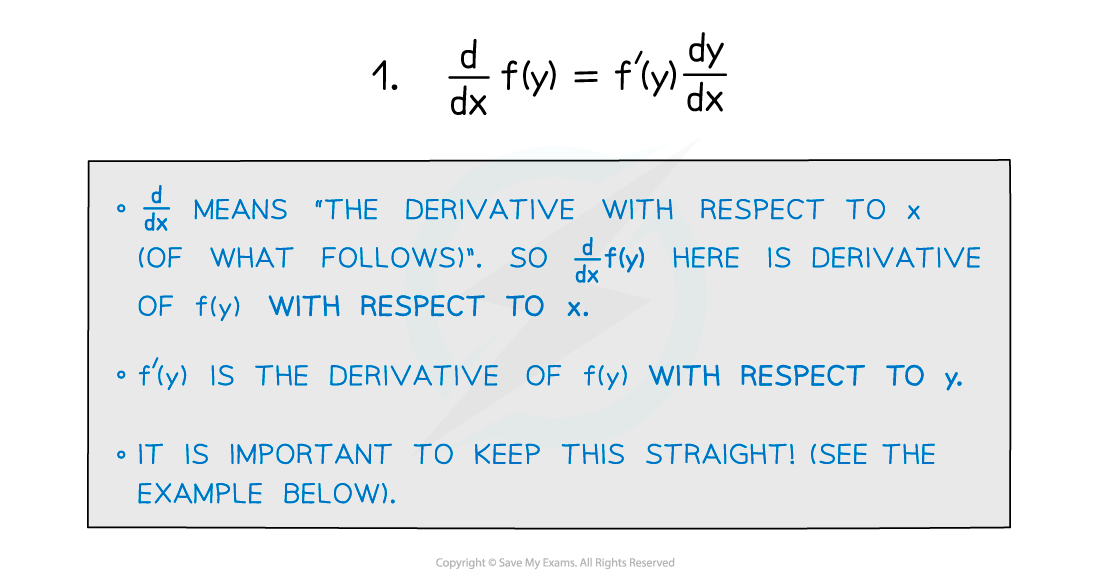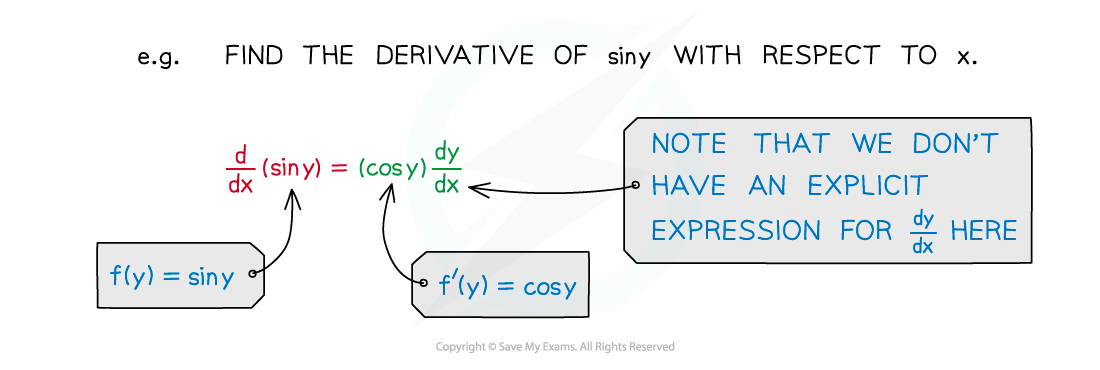• Combining this with the product rule gives us: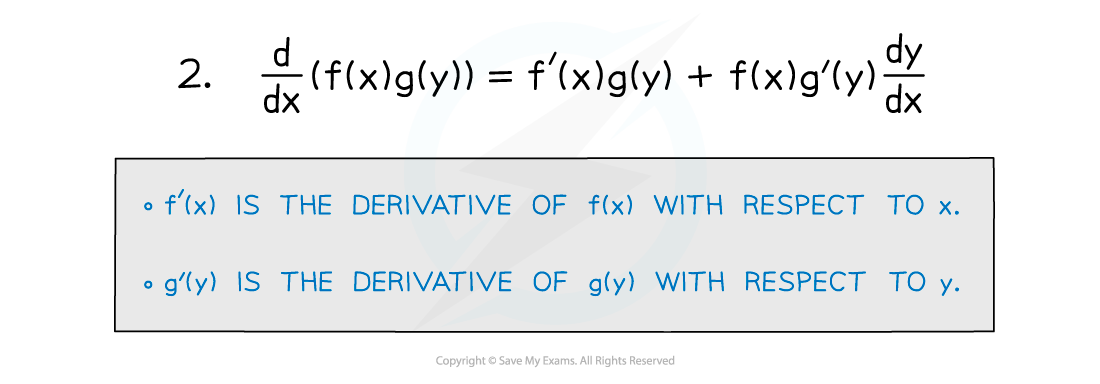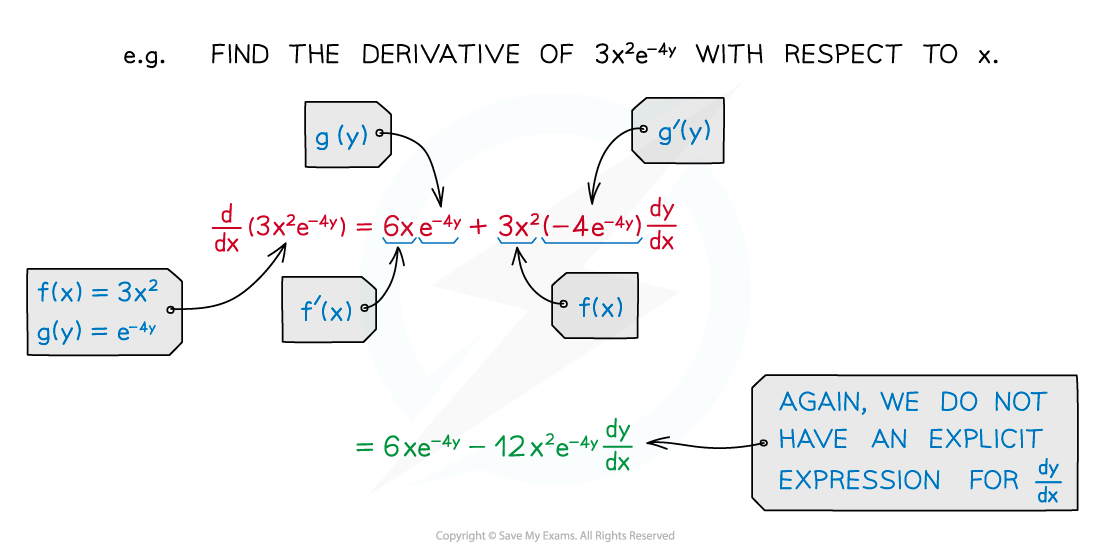• These two special cases are especially useful: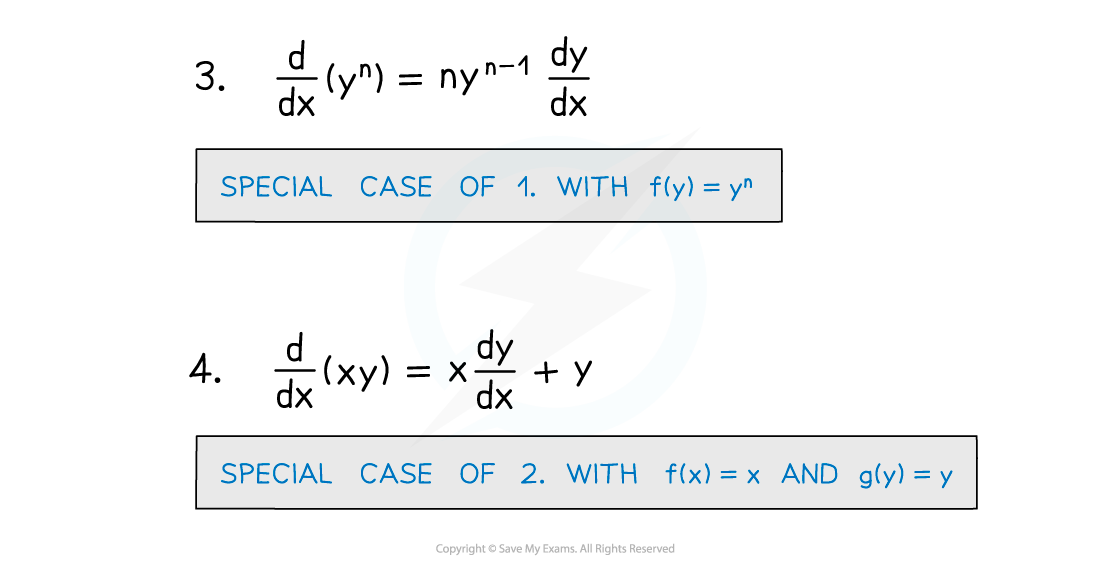• When x and y are connected in an equation you can differentiate both sides with respect to x and rearrange to find a formula (usually in terms of x and y ) for dy/dx
• Note that dy/dx is a single algebraic object
• When rearranging do not treat dy/dx as a fraction
• Especially do not try to separate dy and dx and treat them as algebraic objects on their own!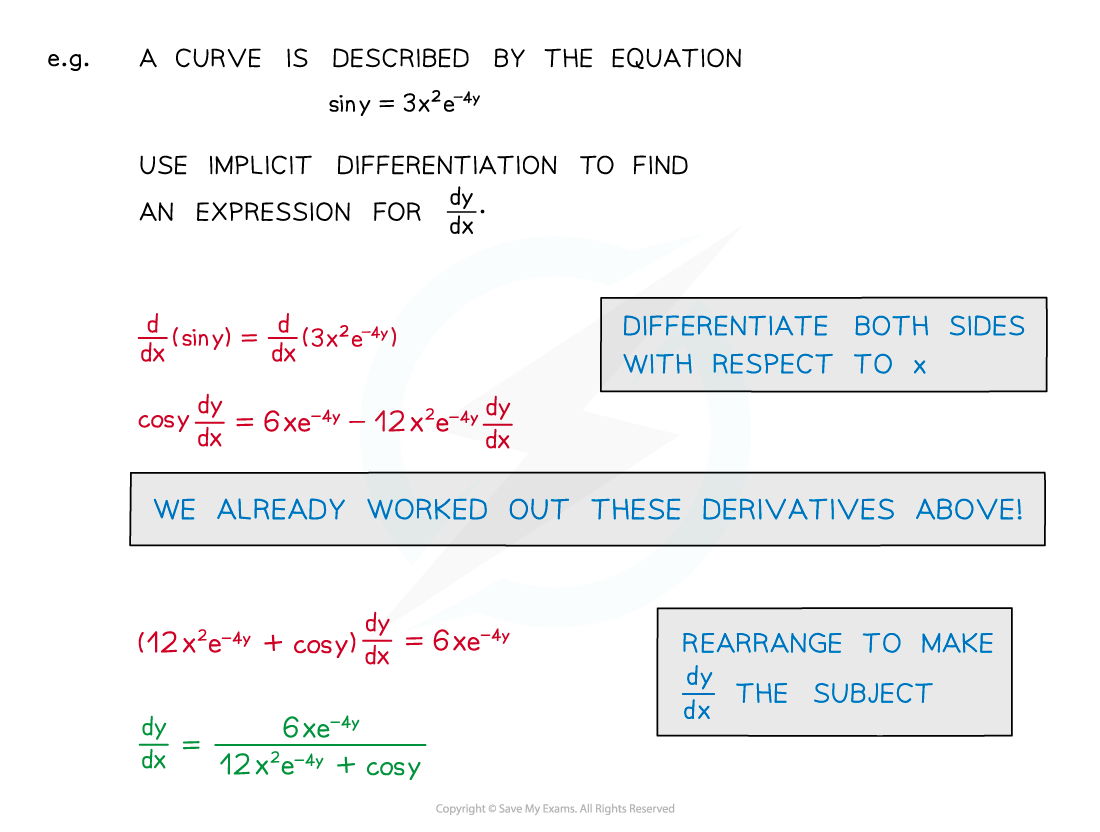#### Exam Tip

• When using implicit differentiation you will not always be able to write dy/dx simply as a function of x.
• However, this does not stop you from answering questions involving the derivative.

#### Worked Example